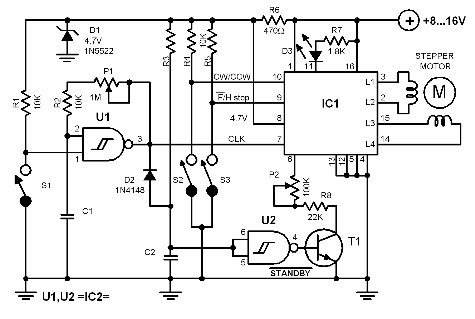# Bipolar Step Motor Controller Circuit Diagram

Bipolar Step Motor Controller Circuit By using a well designed IC like MC3479, it is very easy to control a bipolar two phase step motor. The featured circuit shows how it is done. The maximum stator current can be set by the resistor R8 and P2. This resistor value is however only valid when the output transistors are not in saturated state. To find this value use the following formula:Bipolar Step Motor Controller Circuit Diagram

### Bipolar Step Motor Controller Circuit

By using a well designed IC like MC3479, it is very easy to control a bipolar two phase step motor. The featured circuit shows how it is done.

The maximum stator current can be set by the resistor R8 and P2. This resistor value is however only valid when the output transistors are not in saturated state.

To find this value use the following formula:

I = Vs-0.7/( R*0.86)

The power supply depends on the stator coils and can be between 7.2V and 16.5V. The four ground terminals of the IC must be soldered to the ground plate.

The four inputs are TTL and CMOS compatible. To control the stepmotor correctly, the following must be taken into consideration.

1. The logic at pin 10 determines the direction of the motor's rotation.
2. Pin 9 (FH) determines whether the motor moves with half or full step.
3. The clock pulse must be at least 10S long.
4. The maximum clock frequency is 50 kHz.

The gates U1 and U2 function as a clock generator that can control the stepmotor driver stage without using a computer.

Potentiometer P1 varies the output frequency of this generator and the motor's speed as a result. Of course the stepmotor can be controlled through a computer by using an appropriate program.

The current consumption depends entirely on the type of the stepmotor. The IC itself consumes a maximum of 70 mA.